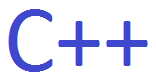﻿ How to Loop Through a Vector Array in C++ ﻿# How to Loop Through a Vector Array in C++In this article, we show how to loop through all items of a vector array in C++.

A vector array is like a regular array but is adaptable, in that new elements can be added or removed from the array.

So let's say we have a vector array created in our code with items in it. How can we then loop through all these items to do something such as display each item in the vector array?

To do this, we use a for loop and use the .at() function to get the value contained in the specified element number. In the at() function, we pass in the element number and this function returns the value of the specified element.

Below we created a vector array that has the elements, 1,4,7,9.

The code below loops through all the elements of this vector array and displays them.

In order to create a vector array and work with vectors in C++, you must include the vector class.

We create a vector that has 4 elements: 1,4,7, and 9.

We then create a for loop that will go through each of the elements in the vector array.

Vector arrays, just like regular arrays, start at element 0. So we know this is the starting point of the array.

How we know how many elements there are in the array is by using the size() function. The size() function will let us know how many elements are in the array, in this case, 4 elements. So we know where we stop.

We then display the vector elements along with their values.

The above code will have the following output shown below.

So this is how we can loop through all items in a vector array in C++.

Related Resources

How to Write to a File in C++